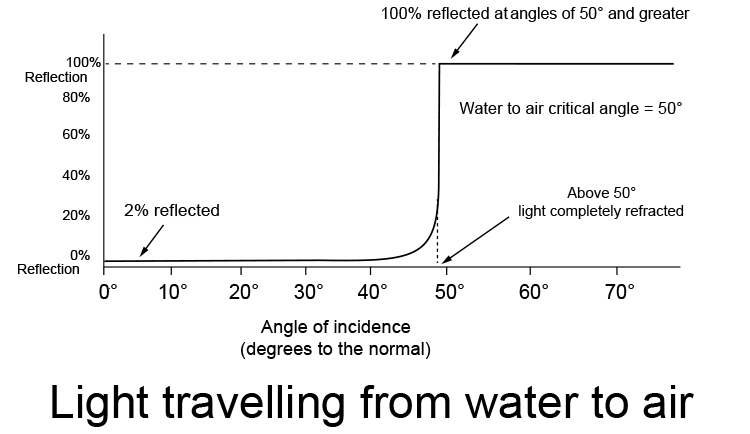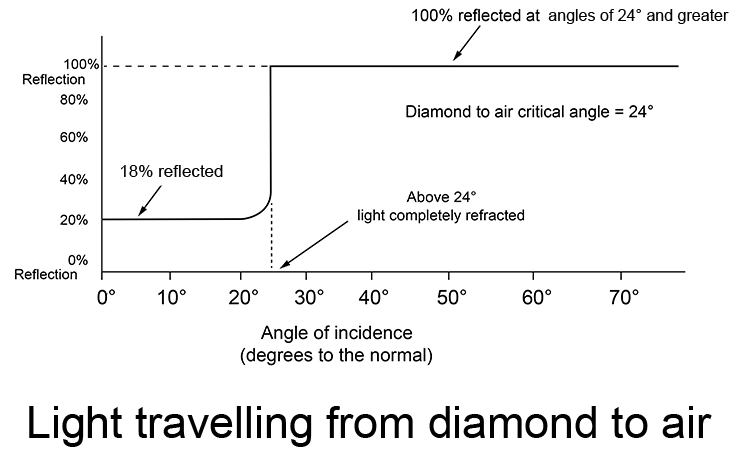# Why total internal reflection is always slow to fast

You don't need the following in an exam, it is just interesting to know.

Total internal reflection only occurs when travelling from a slow medium to a fast medium, and when the ray of light going towards the boundary is greater than the critical angle.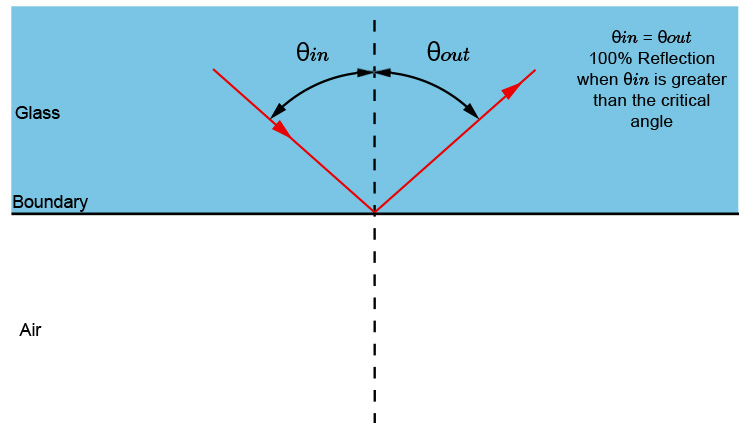They call this "Total internal reflection". So does this mean that at other angles the ray of light is not all reflected?

In nearly all questions in exams at basic level the reflection is not taken into account until total internal reflection occurs. In reality, depending on the angle different amounts of refraction occur as follows: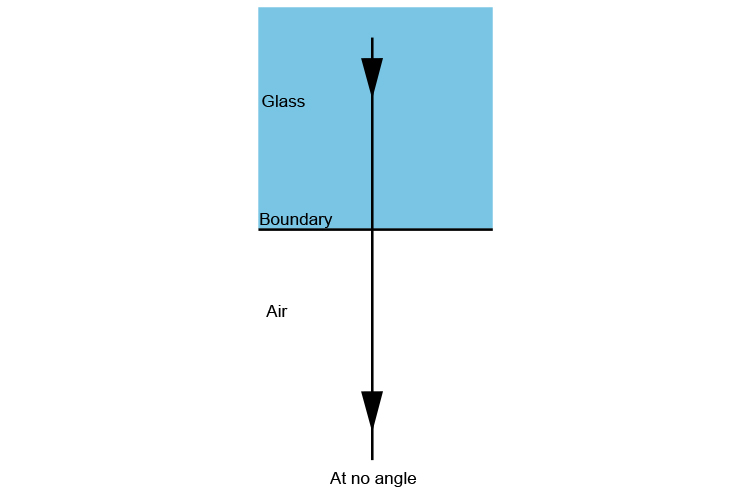When the light is exactly in line with the normal line the light beam that passes into the air is very bright but 3% is reflected backwards into the glass.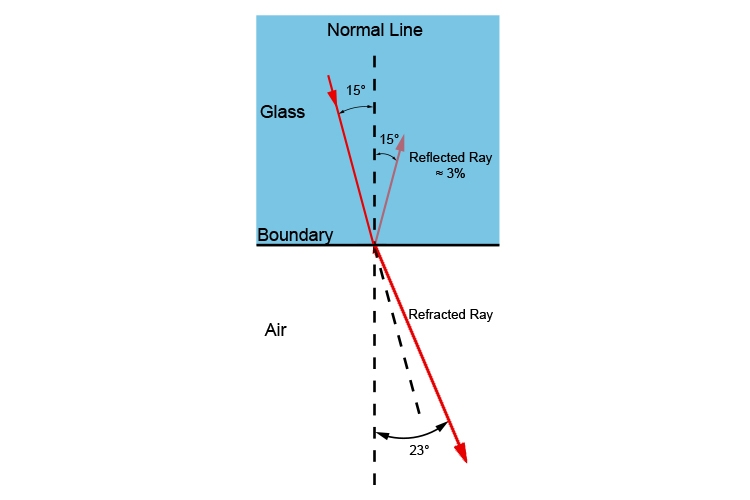At 15° angle of incidence, there is a reflected ray of 3% strength of the original ray leaving 97% to pass into the air.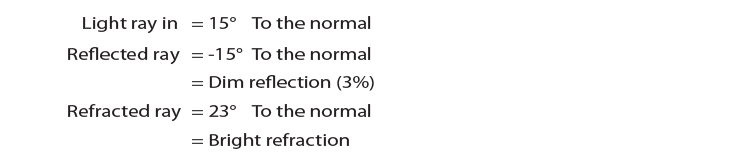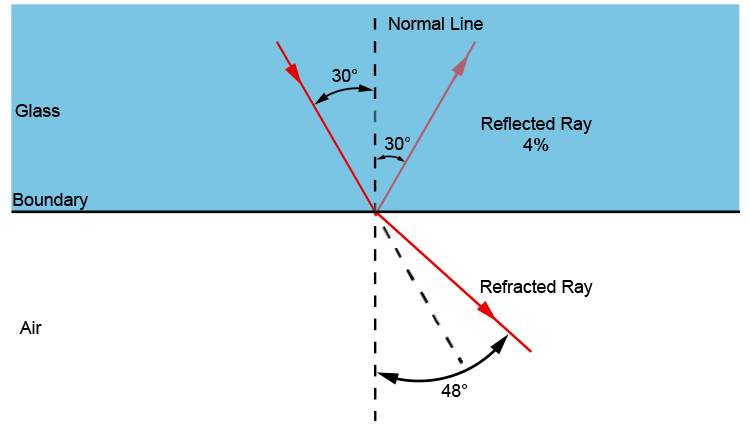At 30° incidence there is a reflected ray of 4% strength.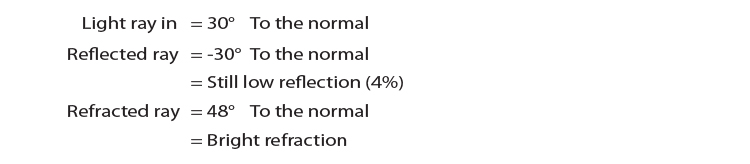At 40° incidence there is a reflected ray of 25% strength leaving 75% of the strength of the ray to pass into the air.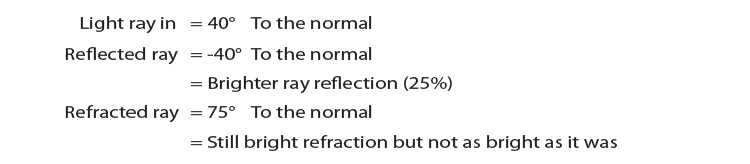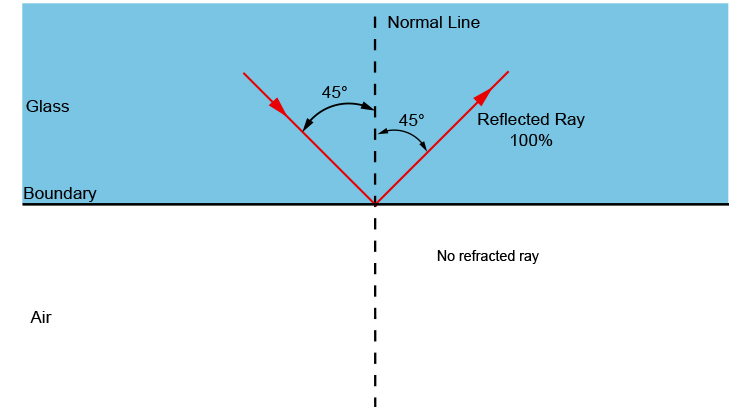When total internal reflection occurs 100% gets reflected.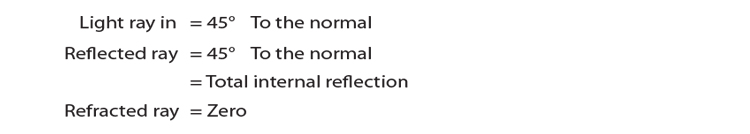(Ray of light going towards the boundary is greater than the critical angle)

If you were to draw a graph of the amount of light reflected at the boundary when light travels from glass to air you would get the following: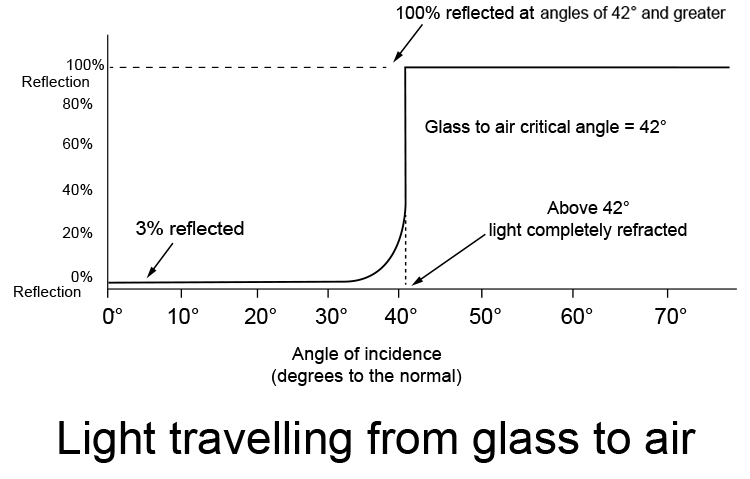If we plot a similar graph for water to air, and diamond to air, you get the following graphs.